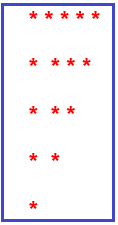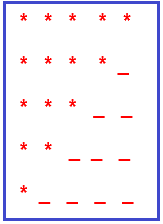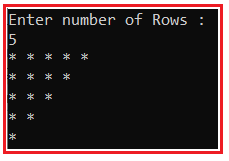# Downward Triangle Star Pattern Program in C#

## Downward Triangle Star Pattern Program in C# with Examples

In this article, I am going to discuss How to implement the Downward Right-Angle Triangle Star Pattern Program in C# with Examples. Please read our previous article where we discussed discuss Mirrored Right Angle Triangle Star Pattern Program in C#. Please have a look at the below image which shows the Downward Triangle Star Pattern.##### Understanding the Downward Triangle Star Pattern

If we want to create a downward triangle star pattern with five rows, then

1. In the first row, we have 5 stars and 0 blank spaces.
2. In the second row, we have 4 stars and 1 blank space.
3. In the third row, we have 3 stars and 2 blank spaces.
4. In the fourth row, we have 2 stars and 3 blank spaces.
5. In the fifth row, we have 1 star and 4 blank spaces as shown in the below image.1. From the above pattern, it is clear that at each row number of stars is decreasing -1 at each row.
2. For example, the 1st row has 5 stars, the 2nd row has 4 stars and the 3rd row has 3 stars, and so on.
3. From the above pattern, it is clear that at each row number of blank spaces is increasing +1 at each row. For example, the 1st row has 0 space, the 2nd row has 1 space, the 3rd row has 2 spaces, and so on.
##### Calculate the number of stars in each row

Suppose if the number of rows is 5 then

1. In the first row, we have 5(equal to row) stars.
2. In the second row, we have 4 (row-1) stars.
3. In the third row, we have 3 (row-2) stars.
4. In the fourth row, we have 2 (row -3) stars.
5. In the fifth row, we have 1 (row – 4) star.
##### How to Implement Downward Triangle Star Pattern Program in C#?

To solve the above pattern,

1. We are going to use two for loops. One outer for loop and one inner for loop.
2. The outer for loop will be used to handle the rows one by one and the two inner for loop will be used to handle the columns one by one.
3. The inner loop will be used to print the stars according to row and the outer loop job is to go on a new line after printing the current row stars.
##### C# Program to Print Downward Triangle Star Pattern

The following C# Program will allow the user to input the number of rows and then print a downward right-angle triangle star pattern on the console

```using System;
public class DownwardTriangleStarPattern
{
public static void Main()
{
Console.WriteLine("Enter number of Rows :");
for (int i = rows - 1; i >= 0; i--)
{
//j<=i  //let rows = 5
/* first row (0 to 5) = 5 stars
* second row (0 to 4) = 4 stars
* third row (0 to 3) = 3 stars
* and so on
*/
//calculate stars for each row one by one
for (int j = 0; j <= i; j++)
{
Console.Write("* ");
}
Console.WriteLine();
}